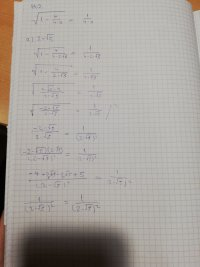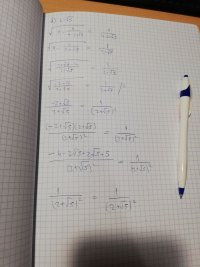How did the solution exclude one possibility?

Harry_the_cat

Elite Member
By definition, the squareroot of anything is non-negative. ie >=0. So the RHS must be non-negative, ie x <= 4.

2 + sqrt(5) > 4, so if you If you sub 2 + sqrt(5) into the RHS, the RHS will be negative.

Jomo

Elite Member
I would NOT find the domain. If you insist on doing it that way, then as Harry-the-cat pointed out you must also include in your domain that the right hand side must be positive. That is that 4-x > 0 or that x<4.
In this problem, I would simply solve the problem and then check my answer to see if any are erroneous.

Also stop using a calculator to see what 2+ sqrt(5) lies between.
We know that 2=sqrt(4) < sqrt(5) < sqrt(9) = 3. So sqrt(5) lies between 2 and 3. Hence 4 = 2+2 < 2 + sqrt(5) < 2+3 = 5. So yes, sqrt(5)>4!

Loki123

Junior Member
By definition, the squareroot of anything is non-negative. ie >=0. So the RHS must be non-negative, ie x <= 4.

2 + sqrt(5) > 4, so if you If you sub 2 + sqrt(5) into the RHS, the RHS will be negative.
What is RHS?

Loki123

Junior Member
I would NOT find the domain. If you insist on doing it that way, then as Harry-the-cat pointed out you must also include in your domain that the right hand side must be positive. That is that 4-x > 0 or that x<4.
In this problem, I would simply solve the problem and then check my answer to see if any are erroneous.

Also stop using a calculator to see what 2+ sqrt(5) lies between.
We know that 2=sqrt(4) < sqrt(5) < sqrt(9) = 3. So sqrt(5) lies between 2 and 3. Hence 4 = 2+2 < 2 + sqrt(5) < 2+3 = 5. So yes, sqrt(5)>4!
We were taught to find the domain which is why I am trying to do it that way. I tried to just check the answers, but they both ended up working. Here is my work on that:I know I should have rationalized them and, therefore, eliminated the square roots from the denominator, but I pretty sure that doesn't change anything. I probably went somewhere wrong, however, I checked it multiple times and couldn't find out where.

Staff member

JeffM

Elite Member
I am going to follow what you were told to do.

$\sqrt{1 - \dfrac{4}{4 - x}} = \dfrac{4}{4 - x}.$
Obviously, if 4 - x is a denominator, then x cannot equal 4. Moreover, if x is greater than 4, then the right hand side of the equation is negative, but a square root is necessarily non-negative. Therefore, x must be less than 4.

$\sqrt{1 - \dfrac{4}{4 - x}} = \dfrac{4}{4 - x} \implies 1 - \dfrac{4}{4 - x} = \dfrac{16}{(4 - x)^2} \implies (4 - x)^2 - 4(4 - x) = 16 \implies \\ 16 - 8x + x^2 - 16 + 4x = 16 \implies x^2 - 4x - 16 = 0 \implies x = \dfrac{4 \pm \sqrt{(-4)^2 - 4(1)(-16)}}{2 * 1} \implies \\ x= \dfrac{4 \pm {16 + 64}}{2} = \dfrac{4 \pm {80}}{2} = \dfrac{4 \pm 2\sqrt {5}}{2} = 2 \pm \sqrt{5}.$
This is what you got. But you

However, we previously determined that x < 4. And obviously

$4 < 5 \implies 2 \le \sqrt{5} \implies 2 + \sqrt{5} > 4 \implies x \ne 2 + \sqrt{5}.$
Therefore that answer is not relevant based on the domain restriction. Our brilliant resident feline explained that way back in post 2.

•Subhotosh Khan

Jomo

Elite Member
When you are checking the solution you want to verify that the left hand side really does equal 1/(2-sqrt(5)). You should not square both sides as that is what causes trouble.

For example if you have x=2, then x^2 = 4 so x = +/- 2. But that is absurd as you were told x=2!

Also, since you are are checking to see if the left hand side equals the right hand you should NOT use equal signs! Equals signs are used when you know that what is on side of the equal sign equals what is on the other side of the equal sign. The reason that you squared bot sides is because you felt that both sides are equal. You should put a question mark over the equal sign.

topsquark

Senior Member
@Loki123,,, Please double check what class you are taking!

-Dan

JeffM

Elite Member
@Loki123,,, Please double check what class you are taking!

-Dan
Maybe he thought this was “Different Equations”?

Loki123

Junior Member
@Loki123,,, Please double check what class you are taking!

-Dan
I am taking mathematics... The education system is different around the world, please keep that in mind.

topsquark

Senior Member
I am taking mathematics... The education system is different around the world, please keep that in mind.
Please understand that I'm no longer getting on your case but is your class actually named "Mathematics?" What is the exact name of the class?

-Dan

Loki123

Junior Member
Please understand that I'm no longer getting on your case but is your class actually named "Mathematics?" What is the exact name of the class?

-Dan
Mathematics. But we call it just math.To be honest, I don't like how the education system is where I am, I think multiple classes on different subjects relating to mathematics would have been better, but I can't really do anything about it other than learn.

•topsquark

HallsofIvy

Elite Member
"Mathematics" includess a huge number of subdivisions:
arithmetic
algebra
geometry
trigonometry
pre-calculus
calculus
differential equations
differential geometry
and more.

We were hoping for a more specific label than just "mathematics" so we could better see exactly what it is you are trying to do.

Loki123

Junior Member
Not quite, Loki. In your check below, that first equation is not true (as the cat had noted, in post #2).

View attachment 29054

It says that 4.2361 equals -4.2361 (rounded).I was trying to see if it's true by simplifying it... I got the same result on both side at the end, where did I make a mistake for that to happen if the equation is not true?

Otis

Elite Member
where did I make a mistake
You'd started the check with a false equation. See post #7. That equation is not true.Loki123

Junior Member
You'd started the check with a false equation. See post #7. That equation is not true.Perhaps I am not explaining myself the best. Imagine if the = wasn't there. I didn't move anything from one side to the other so it makes no difference. So say I was just repeating same actions on two problems, how did I in the end get them to equal the same thing if they weren't the same from the start?

Harry_the_cat

Elite Member
Imagine starting with
-2 = 2 (which is clearly not true).

If you square both sides, you get
4 = 4 (which is true).

That is effectively what you have done!

•Subhotosh Khan